Mobile QR Code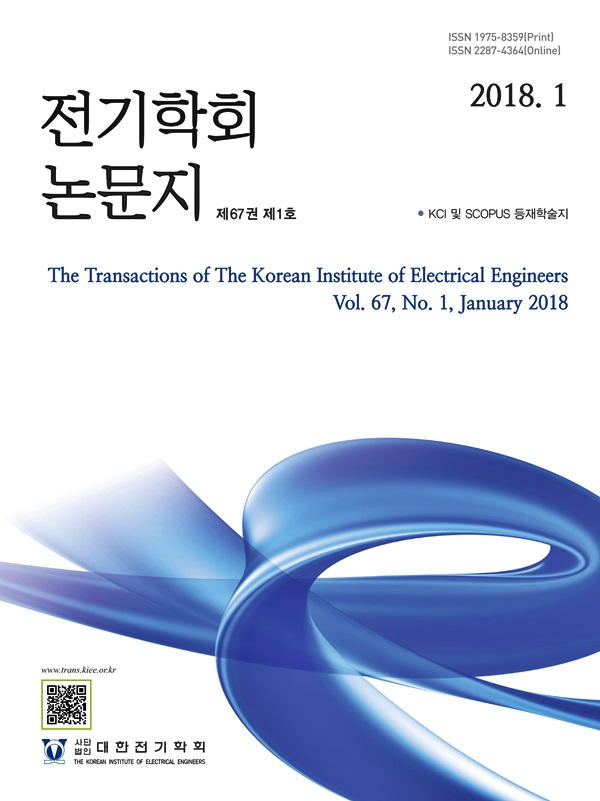#### The Transactions of the Korean Institute of Electrical Engineers

##### ISO Journal TitleTrans. Korean. Inst. Elect. Eng.

1. (Electrical & Electronics and Communication Eng. Dep., Koreatech University, KoreaDep. of Electrical Eng., Assiut University, Assiut, Egypt.

Email :

ahmed21490@koreatech.ac.kr

)

Isolated phase shift PWM converter, ZVS, high frequency magnetic parts, Kool Mu core, power loss analysis, PSIM.

## 1. Introduction

The increasing use of universal technological devices, which are one of the main sources of electrical energy consumption, has resulted from the broad deployment of 5G technology in communications and telecom networks. The source AC voltage must be rectified because these electrical equipment normally operate on DC power. Traditional AC-DC rectifiers can power these devices, but their circuit performance and power efficiency in high-power density applications limits their use (1), thus they must be improved to be more energy efficient. As a result, a highly efficient AC-DC power supply with a high power factor has been changed recently.

Figure 1 depicts the overall structure of an AC-DC telecom power supply, which consists of two stages: a power factor correction (PFC) stage and a DC-DC output converter stage, which is the best option for high power performance and good energy quality (2), (3). For telecom power applications, the second stage of the telecom power supply is implemented to regulate the DC bus voltage to the desired load voltage (typically 45~63V) for the telecom power server (4), (5).

Fig. 1. The Structure of industrial two-stage telecom power supply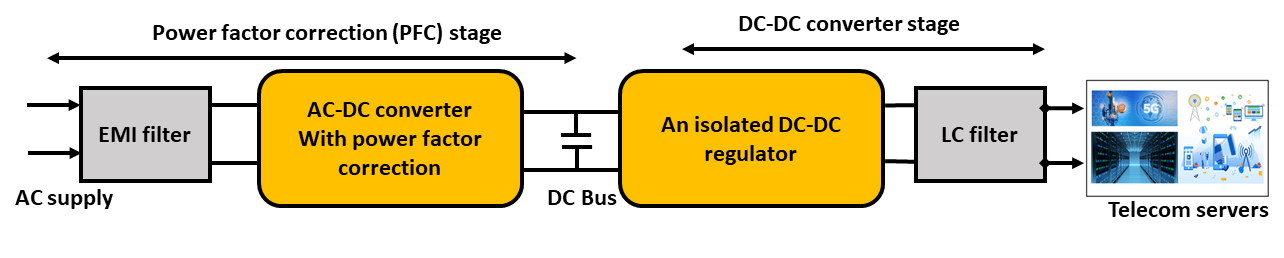An effective technique must be chosen at this stage to offer high power density conversion with high efficiency and low voltage, current and thermal stresses in the converter different power components. The DC-DC converter stage can be constructed as an isolated (6-9) or non-isolated (10) converter depending on the power application.

The isolated converter is more extensively utilized in telecom power applications because it is better suited to providing insulation and protection for connecting loads than the non-isolated converter (11), (12). However, the Choosing of the suitable switching schematics for the converter to reduce the stress on the switches during converter operation is one of the most critical concerns in DC-DC converter circuit design. Therefore, an isolated DC-DC converters based on phase-shift PWM switching technique have recently become one of the most widely used techniques due to their ability to reduce switching losses and provide better regulation over a wide load range, particularly when zero-voltage switching (ZVS) operation is available for the converter switches. It is also possible to have high power density conversion with minimal voltage stress and modest switching losses by adopting ZVS operation (13), (14). Another essential consideration in the design of isolated phase shift PWM DC-DC converters with ZVS is the magentic power components design and manufacturing including the transformer and resonant inductor design and selection for providing ZVS over a wide load range to minimize switching and conduction losses (15).

In this paper, the design considerations and manufacturing techniques for the high frequency transformer and resonant inductor for the isolated phase shift PWM DC-DC telecom converter stage are proposed to offer conversion with ZVS and low thermal stresses, low conduction and switching losses. also, an output inductor filter to maintain output voltage ripple at the specified values is designed and manufactured. The performance of the 2kW DC-DC converter stage with using the optimized design components are simulated using the PSIM simulation to investigate the converter performance. Furthermore, the printed circuit board (PCB) with the power rating of 2kW is designed and fabricated to experimentally testing the designed DC-DC converter efficiency and to calculate the power loss budgets in the converter different power components.

The remainder of this paper is structured as follows: The optimal design analysis and manufacturing of the magnetic power components of the employed DC-DC converter stage are described in Section 2. The PSIM modeling and the simulation results are presented in Section 3. The PCB design and experimental results are shown in Section 4. Section 5 is the paper's conclusion.

## 2. Phase shift PWM ZVS DC-DC converter stage design

Figure 2 depicts the schematic circuit of the used telecom

phase shift PWM ZVS DC-DC converter stage, which consists of three major components: the full-bridge switching circuit on the primary side with phase shift PWM and ZVS technique through tuning the resonant inductor Lr to exhaust the energy of the switches parasitic capacitance at the moment of switching, which offers current flow with zero voltage biasing, reducing switching losses and increasing conversion efficiency The synchronous rectifier on the secondary side uses power Mosfets to rectify the HF transformer's output voltage, and the output LC filter to adjust the load voltage, current ripple contents to the specified values.

The following subsections outline the optimal design analysis and manufacturing steps for the HF transformer, resonant inductor, and load filter inductor. The design specifications of the employed phase shift PWM ZVS DC-DC converter based on LCU+ telecom company for the 5G telecom power server are shown in Table 1.

Table 1. Target converter design specifications

 Parameter Value Unit Bus voltage (Vbus) 400 (320-410) V Load voltage (Vo) 54(45-63) V Output voltage ripple 200 mV Rated power (Po) 2000 W Switching frequency 100 kHz Target efficiency 95% Load ripple current 15%

### 2.1 HF transformer design and manufacturing

The effective (operating) duty cycle to calculate the transformer turns ratio, the magnetization inductance to realize the ZVS, transformer core shape, size, and material to reduce oscillations, number of magnetics, and eliminate eddy currents, and high dv/dt to withstand overshoots and high voltage stress in the primary side are the key parameters that must be considered in the high-frequency transformer's design for DC-DC converters.

As a result, the design and manufacturing procedure for high-frequency transformers for high-power density DC-DC converters can be summarized in four steps:

1. Transformer turns ratio (a) is calculated based on maximum operating duty cycle (Dmax) at the minimum bus voltage rating of the converter (Vbus min) as follow:

##### (1)
$a=\dfrac{N_{P}}{N_{S}}=\dfrac{V_{P}}{V_{S}}$

Let Dmax about 70% and Vbus min about 320V, the transformer turns ratio can be calculated as:

##### (2)
$a=\dfrac{V_{P}}{V_{S}}=\dfrac{\left(V_{bus\min}-2V_{d}\right)D_{\max}}{V_{o}+V_{d}}=4.1$

where Vd is the primary Mosfet switch voltage drop and it is assumed to be 0.5V in calculations.

Let a=5, the effective operating duty cycle (Deff) can be calculated as:

##### (3)
$D_{eff}=\dfrac{\left(V_{o}+V_{d}\right)a}{\left(V_{bus}-2V_{d}\right)}=0.675$

2. Transformer magnetizing inductance (Lm) is calculated using the maximum magnetizing inductance to achieve ZVS, as shown in (15), (16).

##### (4)
$L_{m}=\dfrac{T_{dead}aV_{o\min}}{C_{HB}V_{bus\min}}*\left(\dfrac{T_{s\min}}{4}-\dfrac{T_{dead}}{2}\right)$

where CHB denotes the full equivalent capacitance of the primary H bridge, which can be found on the primary switch data sheet. Ts min is the required minimum switching frequency's minimum switching time; Tdead is the PWM dead time, which can be calculated using the previously stated effective duty ratio.

3. The size, shape, and material of the transformer core are all determined by factors such as efficiency, temperature rise, operating frequency, eddy currents, and core losses. For high-frequency applications, iron powder cores, amorphous steel cores, and ferrite cores can all be used (10kHz-3MHz). The ferrite cores, on the other hand, are effective eddy current insulators with a very low core loss. When choosing a core, you can begin with a core-weight (We), core effective volume (Ve), or core effective area (Ae) based on the transfer power and switching frequency, and then select the best-suited core from there.

The TDK supplier's ferrite core with a PQ shape and effective area of about 200mm2 is excellent for the DC-DC converter switching frequency (fsw=100kHz) and high-power density in this work. Generally, for acceptable core losses, the transformer magnetic flux should be kept low (0.1-0.4)Tesla. As a result, we limit the maximum magnetic flux (Bmax) to 0.35Tesla, and the number of primary turns (Np) is calculated as follows:

##### (5)
$N_{P}=\dfrac{V_{bus}× D_{eff}}{2× B_{\max}× A_{e}× f_{SW}}=19.10Turns$

Using an HF transformer with a primary winding of 20 turns, the operating maximum magnetic flux is calculated to be around 0.336Tesla.

4. The core losses, primary cupper losses, and secondary cupper losses are the three main losses in the transformer that must be estimated.

For the ferrite cores, the transformer core losses can be calculated as (19)

##### (6)
$P_{trcoreloss}=0.036.\left(\dfrac{f_{sw}}{10^{3}}\right)^{1.64}.\left(10.B_{\max}\right)^{2.68}.V_{e}.10^{3}$

The cupper losses due to the primary winding resistance (Rp) and the primary winding rms current (Ip) can be computed as

##### (7)
$I_{p}=\dfrac{I_{o}}{2}\times a$

##### (8)
$P_{ploss}=I_{p}^{2}.R_{p}$

The cupper losses due to secondary winding resistance (Rs) and the secondary winding RMS current (Is) can be computed as

##### (9)
$I_{s}=\dfrac{I_{o}}{2}\times\sqrt{2D_{eff}}$

##### (10)
$P_{sloss}=2.I_{s}^{2}.R_{s}$

### 2.2 Load (output) filter inductor design

The filter inductance value Lf is calculated using the specified ripple current ILf expressed as a percentage of the full load current (15%) as provided in Table 4's design parameters. The value of the filter inductance can be estimated using KVL in the secondary circuit of the DC-DC converter stage. The value of the filter inductance can be calculated as

##### (11)
$L_{f}=\dfrac{V_{load}\left(1- D_{eff}\right)}{∆I_{Lf}* f_{SW}}$

The maximum peak current (ILf pk) of a load filter inductor can be computed as

##### (12)
$I_{Lfpk}= I_{load}+\dfrac{∆I_{Lf}}{2}$

Using the LI2 method to select the inductor core from the Kool Mu chart as shown in Fig. 3, the single-core Kool Mu with the part number 77493 was chosen for the load filter inductor design. The number of turns (NLf) to obtain the required inductance value (Lf) can be calculated as:

##### (13)
$N_{Lf}=\sqrt{\dfrac{L_{f}}{A_{L_{\min}}}}$

The copper loss (PLf DCR) of the winding wire due to the inductor DCR can be calculated on a maximum value of the rms inductor filter current (ILf) as given

##### (14)
$I_{Lf}=\sqrt{\left(\dfrac{P_{load}}{V_{load}}\right)^{2}+\left(\dfrac{\triangle I_{Lf}}{\sqrt{3}}\right)^{2}}$

##### (15)
$P_{Lf_{Loss}}=I_{Lf}^{2}\times DCR$

The core losses of the inductor (PLb Core) is calculated using the given formula

##### (16)
$P_{Lfcore}=K_{1}\times F_{sw}^{x}\times B^{y}\times V_{e}\times 10^{-3}$

In this work, in order to design inductor which, withstand the peak current of about (39.80) A at full loading condition of about 2000W, the load filter inductor (Lf) with value of 30uF is manufactured with 16 turns of the 3-wire copper with 1.15mm diameter size around the Kool Mu 77439A9 toroids core which also reduced the equivalent DCR value by increasing the wire cross section area. The manufactured load filter inductor DCR value was experimentally measured, and it was about 0.0029Ω.

### 2.3 Resonant inductor design and manufacturing

The size of the resonant inductor tank is determined by the amount of energy necessary to produce ZVS condition. As a result, the resonant inductance (Lr) and transformer leakage inductance (Llk) inductor values must be able to exhaust the energy provided by the parasitic capacitance of the primary switches (Coss) as well as the energy provided by the transformer winding capacitance (Cw).

##### (17)
$\dfrac{1}{2}I_{P}^{2}\left(L_{r}+L_{lk}\right)\ge\dfrac{4}{3}C_{oss}V_{bus}^{2}+\dfrac{1}{2}C_{w}V_{bus}^{2}$

The leakage inductance of the designed HF transformer is around 1.9uH at the primary, while the transformer winding capacitor is approximately 0.90nF. The resonant inductor to achieve the ZVS in the primary side can be calculated using these parameters.

The peak current of the resonant inductor is calculated as

##### (18)
$I_{Lrpk}=\dfrac{I_{Lfpk}}{a}$

Using the LI2 method to select the inductor core from the Kool Mu chart as shown in Figure 4, the single core Kool Mu with the part number 77120A7 was chosen for the load filter inductor design. The manufactured load filter inductor DCR value was experimentally measured, and it was about 0.013Ω. The number of turns (NLr) to obtain the required inductance value (Lr) can be calculated as:

##### (19)
$N_{Lr}=\sqrt{\dfrac{L_{r}}{A_{L_{\min}}}}$

The core losses of the resonant inductor can be using (16), and the copper loss (PLr DCR) of the winding wire due to the inductor DCR can be calculated on maximum value of the rms inductor filter current (ILr) as given

##### (20)
$I_{Lr}=\dfrac{I_{Lf}}{a}$

##### (21)
$P_{Lr_{Loss}}=I_{Lr}^{2}\times DCR$

In this work, the resonant inductor (Lr) with value of 31uF is manufactured with 22 turns of the single wire copper with 1.15mm diameter size around the Kool Mu 77120A7 toroids core. The manufactured load filter inductor DCR value was experimentally measured, and it was about 0.013Ω.

The designed load filter and resonant inductors specifications for the 2kW isolated phase shift PWM ZVS DC-DC telecom converter stage are summarized in Table 2.

Table 2. specifications of the manufactured inductors

 Parameter Resonant inductor Filter inductor Inductance 31uF 30uF Peak current 7.96A 39.80A Core type Single Kool Mu (77120A7) Single Kool Mu (77439A9) AL 72 ±8%(nH/T2) 135±8%(nH/T2) No. of Turns 22 16 Winding wire Single copper wire 1.15mm 3-Wire copper 1.15mm DCR 0.013Ω 0.0029Ω Ploss (W) 1.815 15.50

### 2.4 Control circuit implementation of the employed converter

Fig. 5. Control circuit structure of the employed phase shift PWM DC-DC converter stage.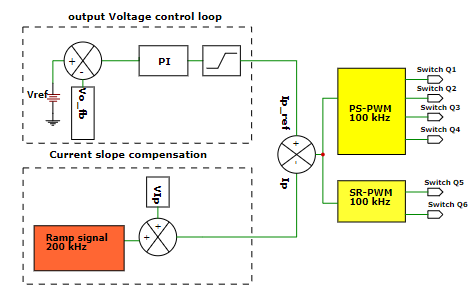Figure 5 depicts the control strategy for the DC-DC converter stage's voltage and current control loops, which is used to control the primary and secondary switches of the phase shift PWM DC-DC converter stage.

The phase shift PWM technique is used to control the whole bridge on the primary side of the DC-DC converter by phase shifting the switching pulses of one half-bridge with respect to the other. The ZVS method is used to achieve high power density efficient conversion at a high switching frequency in this section. Voltage-mode or current-mode control techniques can be used in this section. Because it is more efficient than voltage mode control, current-mode-controlled DC-DC switching is popular. However, when the PWM duty cycle exceeds 50%, the current-mode architecture can become unstable. To alleviate this instability and restore stability across a wide duty-cycle range, the converter's primary current slope compensation approach is used (5), (8).

The primary current and output voltage are the feedback signals in this control system. A current transformer with a 100:1 turns ratio is used to sense the primary current, which is then sampled by a resistor to obtain a primary current signal (VIp). In addition, a load voltage signal (Vo fb) is detected. A 200kHz ramp signal is added to the VIp signal to generate the primary current to compensate for the instability of current waveforms at high duty cycle values (Ip). The output voltage feedback signal (Vo fb) is compared to the appropriate reference value (Vref) and passed to the voltage PI controller to generate the primary current reference value (Ip-ref). The primary current (Ip) is then compared to the reference value (Ip-ref) to generate the duty cycle required for the phase shift PWM (PS-PWM) and synchronous rectifier PWM (SR-PWM) generators to generate the operating pulses for the primary and secondary switches.

## 3. Simulation Results and Discussion

PSIM software is used to investigate the performance of the employed DC-DC converter with the designed power components shown in Table 3.

Table 3. Designed power components

 Component Value/part number Input capacitance 470 uF Primary Mosfets IPW60R070CFD7 Resonant inductor 31 uF H.F transformer Core:PQ40/40, a=20:4:4, Lm=2.8 mH Secondary Mosfets IPP110N20N3 Load inductor 30 uF Load capacitor 470 uF

At a rated input voltage of 400V and full loading condition, the input-output power waveforms are simulated as shown in Fig. 6, it is observed that the designed converter offers full loading power conversion with an efficiency of about 97.20%.

Fig. 6. Input-output power waveforms with rated input voltage and full loading condition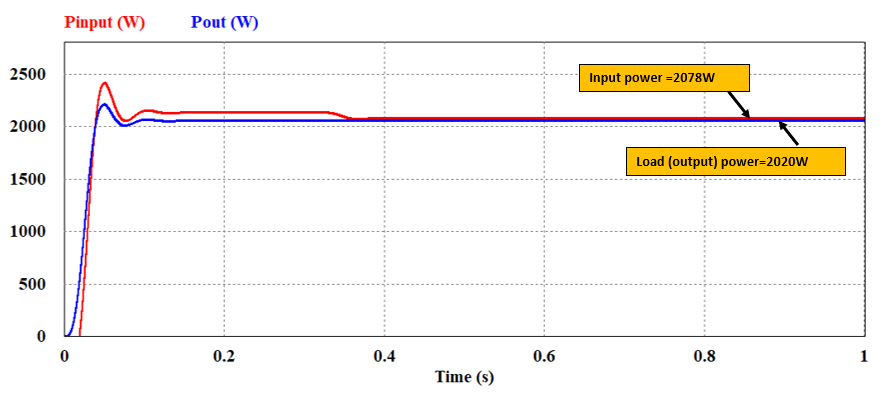The output voltage control loop and the output filter inductor and capacitor are optimally designed to regulate the load voltage and current with the specified ripple contents for the telecom power applications as specified in Table 1. in order to investigate the performance of the designed converter load voltage and current ripple contents, the load voltage and current waveforms are simulated with full loading condition of 2kW as shown in Fig. 7, it is observed that the voltage ripple contents of about 13.5mV, and the current ripple contents of about 20mA which is less than the specified values.

Fig. 7. Full load voltage and current waveforms and ripple contents measurements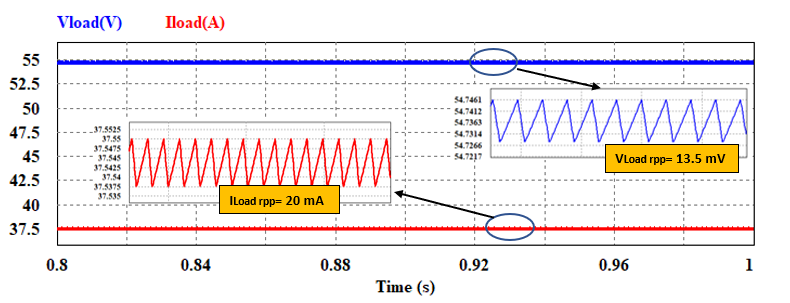The voltage and current of the switches Q1 and Q3 in the two legs of the full-bridge of the primary side of the designed DC-DC converter are shown in Fig. 8, with the current waveforms multiplied by scale 20 for easier viewing. it is observed that, the optimally designed resonant inductor and the proposed phase shift PWM control approach in the converter primary side allow converter operation with ZVS at the two legs of the full-bridge in the converter primary side at full loading condition.

Fig. 8. Switches voltage and current waveforms in the two legs of the primary full bridge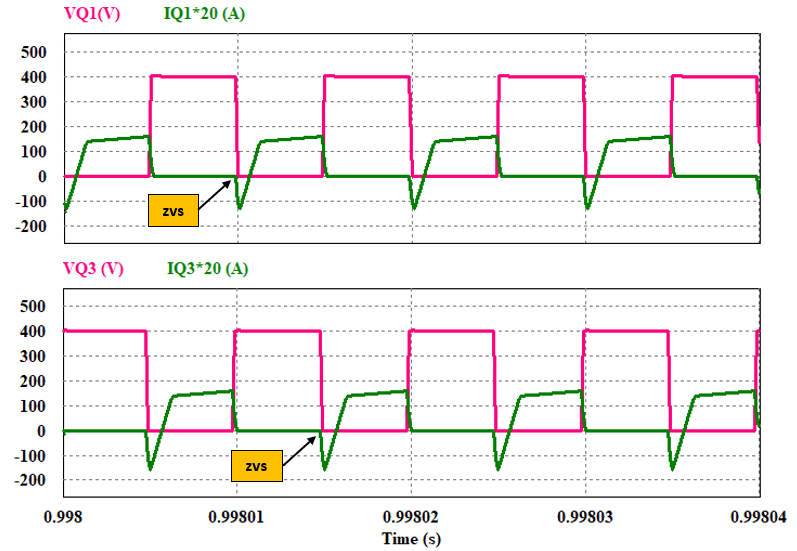## 4. xperimental verification

Fig. 9. The manufactured 2000W PCB for the telecom phase shift PWM DC-DC converter stage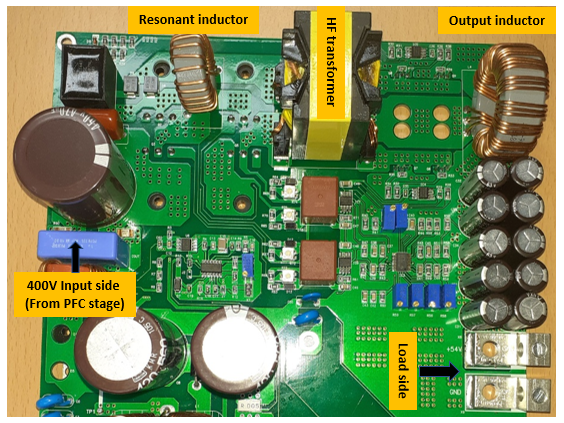Figure 9 shows the printed circuit board (PCB) of the 2kW phase shift PWM DC-DC converter designed using OrCAD capture and PCB design software and manufactured using the optimally designed magnetic power components mentioned in the photograph and the selected power components given in Table 3.

To withstanding the high current at the output side the filter inductor is manufactured using a 3-wire conductor which increases the conductor cross-section area and reduces the equivalent inductor DCR which reduced the inductor power losses. the control circuit of the employed converter is implemented using The UCC 28950 IC from Texas Instruments which provides 4-PSPWM signals with a switching frequency of 100kHz for the primary side switches and 2-SRPWM for synchronous rectification at the secondary side switches, as well as primary current compensation to restore current stability and voltage loop control to adjust the output voltage at the specified value to control the DC-DC converter switches.

With the full loading condition and rated DC bus voltage of 400V, as shown in Fig. 10, the designed voltage control loop and the output filter capacitor regulate the output voltage to about 54.5V with a small voltage ripple of less than 100mV, also, the DC output current to about 38A with ripple current less than 30mA.

Fig. 10. Input-output voltage and output current waveforms at full loading condition.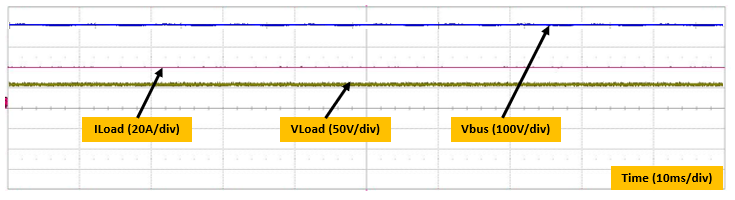The power losses budget in the converter's different power components are measured and depicted as shown in Fig. 12, which shows that the highest power loss budget is contributed in the secondary Mosfets follows by the filter inductor due to the high current stress at full loading condition.

Fig. 11. Conversion efficiency curve of the designed converter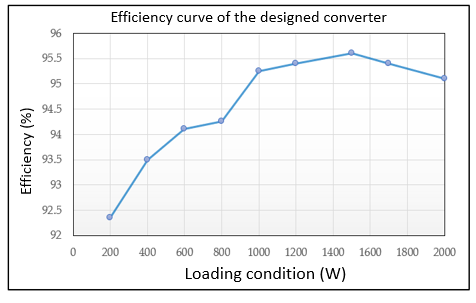The power losses budget in the converter's different power components is measured and depicted as shown in Fig. 12, which shows that the highest power loss budget is contributed in the secondary Mosfets follows by the filter inductor due to the high current stress at full loading condition.

Fig. 12. Fig. 12 Power losses budget in the different power components of the designed converter at full loading condition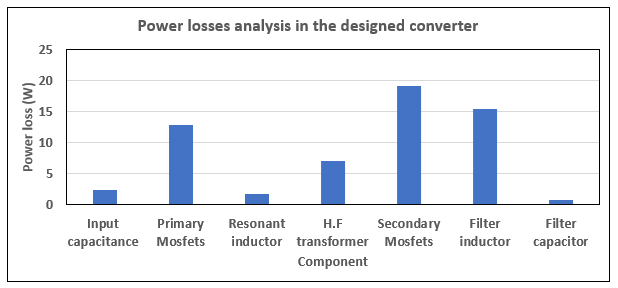## 5. Conclusion

In this paper, the optimal design analysis, considerations, and manufacturing techniques of the magnetic power components in the high power density telecom phase shift PWM DC-DC converter stage is proposed. The designed HF transformer and the resonant inductor maintain the operation of the primary switches with the ZVS technique which reduced the switching power losses and offers high conversion efficiency. An LC filter is utilized on the load side of the DC-DC converter which maintains the voltage and current ripple contents at the standard values required for the telecom power applications. The experimental results show that the designed DC-DC converter stage offers a maximum efficiency of 95.70% at 75% loading conditions and an efficiency of about 95.20% at full loading conditions. The 2kW, 54V phase shift PWM ZVS DC-DC converter stage with an efficiency of more than 95% which is suitable for the telecom power applications is achieved in this work.

### Acknowledgements

This research was funded by grant (2018R1D1A3B0704376413) from National Research Foundation of Korea(NRF)

### References

1
Y. Kim, W. Sung, B. Lee, June 2014, Comparative Performance Analysis of High Density and Efficiency PFC Topologies, in IEEE Trans. on Power Electron., Vol. 29, No. 6, pp. 2666-26792
CA Gallo, FL Tofoli, JA Pinto, Feb 2010, Two-stage isolated switch-mode power supply with high efficiency and high input power factor, IEEE Trans. on Industrial Electron., Vol. 57, No. 11, pp. 3754-663
R Yu, BM Pong, BW Ling, J Lam, Feb 2011, Two-stage optimization method for efficient power converter design including light load operation, IEEE Trans. on Power Electron., Vol. 27, No. 3, pp. 1327-13374
Ahmed H. Okilly, Baek Jeihoon, Aug 2021, Optimal Design Analysis with Simulation and Experimental Performance Investigation of High-Power Density Telecom PFC Converters, Applied Sciences, Vol. 11, No. 17, pp. 79115
AH Okilly, N Kim, J Baek, Jan 2020, Inrush Current Control of High-Power Density DC–DC Converter, Energies, Vol. 13, No. 17, pp. 43016
Haijun Tao, Zhang Guopeng, Zheng Zheng, Du Changshun, Dec 2019, Design of Digital Control System for DC/DC Converter of On-Board Charger, Journal of Advanced Transportation, no. 24673077
Hua Bai, Nie Ziling, Chunting Mi. Chris, Dec 2009, Experimental comparison of traditional phase-shift, dual-phase-shift, and model-based control of isolated bidirectional DC–DC converters, IEEE Trans. Power Electron, Vol. 25, No. 6, pp. 1444-14498
BR. Lin, Dec 2019, Phase-shift pwm converter with wide voltage operation capability, Electronics, Vol. 9, No. 1, pp. 479
P Das, B Laan, SA Mousavi, G. Moschopoulos, Jan 2009, A non-isolated bidirectional ZVS-PWM active clamped DC–DC converter, IEEE trans. on power electron., Vol. 24, No. 2, pp. 553-55810
K Sayed, ZM Ali, M Aldhaifallah, Jan 2020, Phase-Shift PWM-Controlled DC–DC Converter with Secondary-Side Current Doubler Rectifier for On-Board Charger Application, Energies, Vol. 13, No. 9, pp. 229811
IO Lee, GW. Moon, Jun 2012, Phase-shifted PWM converter with a wide ZVS range and reduced circulating current, IEEE Trans. on Power Electron., Vol. 28, No. 2, pp. 908-91912
IH Cho, KM Cho, JW Kim, GW. Moon, Mar 2011, A new phase-shifted full-bridge converter with maximum duty operation for server power system, IEEE trans. on Power Electron., Vol. 26, No. 12, pp. 3491-350013
T Mishima, Y. Koji, 17 Mar 2019, Zero Voltage Soft-Switching Phase-Shift PWM Controlled Three-Level DC-DC Converter for Railway Auxiliary Electric Power Unit, IEEE Applied Power Electronics Conference and Exposition (APEC), IEEE, pp. 1716-172114
Z. Zhao, Q. Xu, Y. Dai, H. Yin, Nov 2018, Analysis, Design, and Implementation of Improved LLC Resonant Transformer for Efficiency Enhancement, Energies, Vol. 11, No. 12, pp. 328815
W.G. Hurley, W.H. Wölfle, 2013, Transformers and Inductors for Power Electronics Theory, Design and Applications, Book, Wiley: London, UK16
S Yue, Q Yang, Y Li, C Zhang, G. Xu, 11 Aug 2017, Core loss calculation of the soft ferrite cores in high frequency transformer under non-sinusoidal excitations, In 20th International Conference on Electrical Machines and Systems (ICEMS), IEEE, pp. 1-5## 저자소개

##### Ahmed H. okilly(아메드 오킬리)In 2012 and 2017, he got his B.Sc. (with honors) and M.Sc. degrees in electrical engineering from Assiut University in Egypt.

He has been an assistant lecturer at the electrical engineering department of Assiut University in Egypt since 2012.

Since 2019, hehas been studying for a Ph.D. at Korea University of Technology and Education in South Korea.

Power electronics, PFC, and VSI circuits, analog and digital control circuits design, power system stability, HVDC and FACTS applications are among his research interests.

In 2006, he got M.S. degrees in electrical engineering from the University of Wisconsin Madison in Madison, WI, and in 2009, he received a Ph.D. in electrical engineering from Texas A&M University in College Station, TX.

He worked for Amotech in Seoul from 1998 to 2000.

From 2000 to 2003, he worked as a Senior Research Engineer at Samsung Electromechanics in Suwon, Korea.

He served as a Principal Engineer at Samsung Advanced Institute of Technology in Youngin, Korea, from 2010 to 2013.

From 2014 to 2016, he worked as a Senior Research Engineer at the Korea Railroad Research Institute in Uiwang, Korea.

He has been an Assistant Professor at Korea University of Technology and Education in Cheonan, Korea, since 2017.

Design and analysis of electrical machines, variable speed drives for traction systems, and novel power conversion topology are two of his current research interests and experiences.